Next: 5.3 Interfaces Up: 5. Contacts and Boundaries Previous: 5.1 Quantities, Segments, Boundaries,

# 5.2 Equation Assembly

MINIMOS-NT solves f = 0 using a damped Newton algorithm:

 -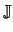k . u = f(xk) (5.1) x* = xk + d . u (5.2)=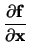(5.3)

Applying the finite boxes discretization scheme to the basic semiconductor equations one gets for each quantity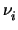located at the grid point i constitutive relations fS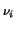of the form

 fS=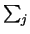F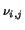+ Gi = 0 (5.4)

where j runs over all neighboring grid points in the same segment, Fis the flux between those grid points, and Gi is the source term at grid point i. (5.4) controls the value of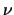. For grid points located at boundaries or interfaces, the situation is different. Despite of general boundary conditions, the two extreme cases Dirichlet (5.5) and Neumann (5.6) are of fundamental importance.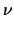= a (5.5) F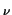= b (5.6)

In case of a Dirichlet condition, (5.4) is not needed as the value ofis determined by (5.5). In case of a Neumann condition (5.6) can be used to calculate (5.4) as fluxes over contacts and interfaces cannot be obtained by discretizing the semiconductor equations.

As is obvious from these considerations, boundary and interface points have to be handled in a different manner compared to points located inside the segments. In a generic device simulator like MINIMOS-NT which can handle an arbitrary number of contacts, segments, and interfaces, efficient handling of these situations is of utmost importance. This is achieved by treating the segments separately from the boundary conditions in such a way that all segment points are treated in the same manner. (5.4) is calculated for all segment points including boundary points. The derivatives of (5.4) for all grid points ( fS) with respect to the solution vector x yield the segment matrixS.

The system matrix(Jacobian) will be assembled from two parts, namely the direct partB (boundary models) and the transformed partS (segment models). The latter will be multiplied by the so called row transformation matrix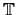B before contributing to the system matrix. The same treatment applies for the right hand side vector b.=B +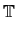B .S (5.7) b = bB +B . bS (5.8)

The direct part of the system matrixB and the row transformation matrixB are set up by the boundary models, while the transformed partS will be handled by the segment models.B is initialized to be the unity matrix. In the actual implementation only entries different to the unity matrix will be stored. Direct matrix multiplication is avoided by assembling the transformation matrix in a pre-pass. The transformation matrix allows for arbitrary linear combination of the equations found inS and is normally extremely sparse with most of the non-zero entries being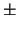1. For the special case with the main-diagonal entry ti, i = 0, the constitutive relation for grid point i will not contribute to the final constitutive relation i. Another special case is ti, j = tj, j = 1 which simply combines rows i and j ofS and adds them to row j ofB.Next: 5.3 Interfaces Up: 5. Contacts and Boundaries Previous: 5.1 Quantities, Segments, Boundaries,
Tibor Grasser
1999-05-31